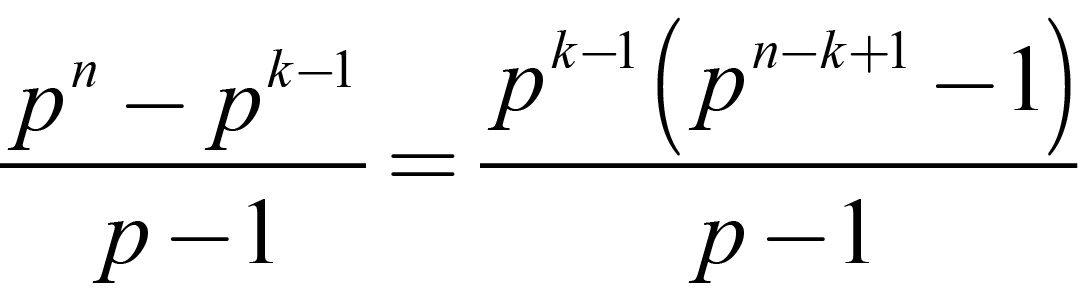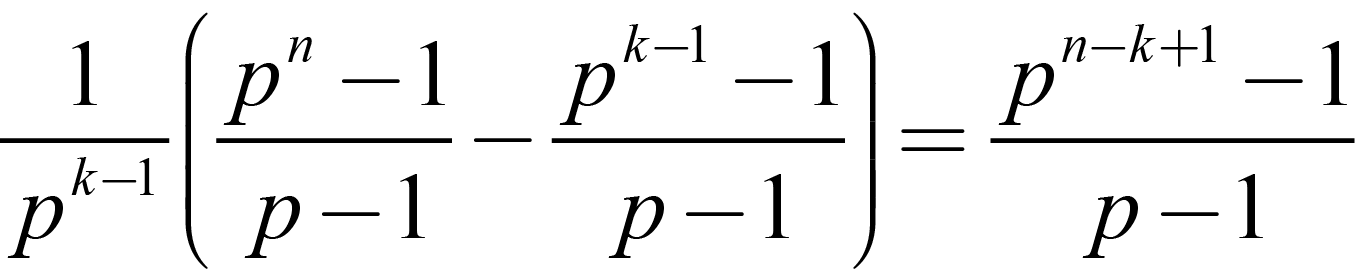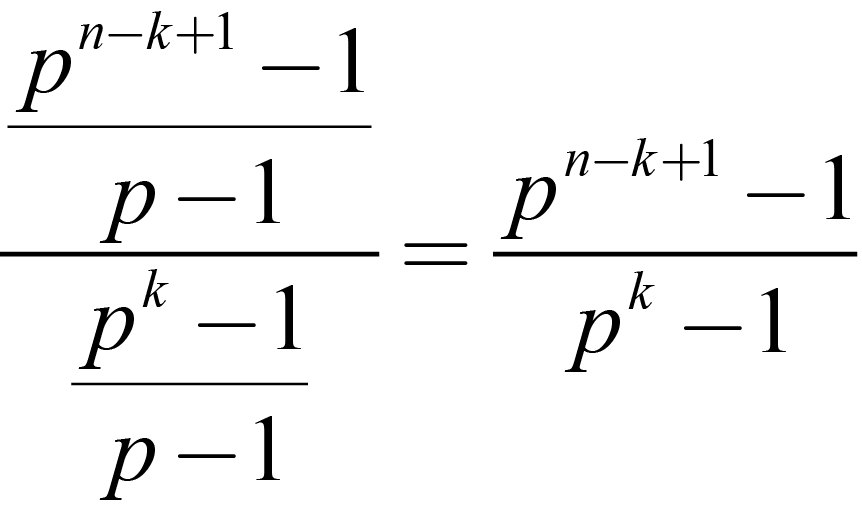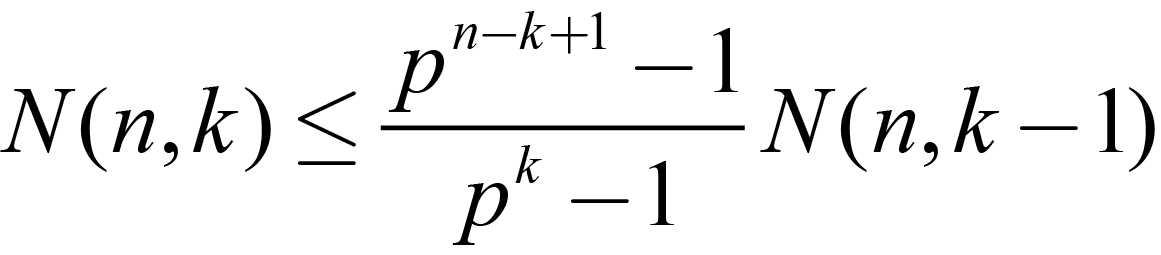None:
Polyps:
Strongs:That Induction Proof N(n,k)

I stated that the formula for the number of additive subgroups of the additive group of a finite field was given by the formula below.Where 'n' is the degree of the cartesian product of the prime subfield of F=GF(p,n) isomorphic to F+ and k is the dimension of the k-fold cartesian product of subgroups isomorphic to the prime subfield of F (which in a k-fold cartesian product form the "group" H in question). The number of such subgroups H of F+ that is given by the formula is exact.

We proceed by induction on k for a given n. I know the case k=1 is correct, as the number of additive groups is simply(1.1)

Since each group is disjoint in elements except for the zero.

Now we start assuming that the case k-1 is correct and we obtain the desired result after a few calculations.

So, given a group H of order pk-1 in G (of order pn) every element not in H may extend the group to one of higher degree. These elements partition into their subgroups; as the extensions that may be possible are similar for every element of each p-order subgroup not in H. So the number of ways I may extend H to some group in G; (each a subgroup Ki) of order pk is in part given by the formula:(1.2)

Which simplifies to:(1.3)

Yet each one of these possible extensions are not unique: for in the extension of order pk there are some few elements in every Ki which generate this group Ki not in H, i.e. these elements are in the set K \ H, (otherwise stated as K - H) the number of extensions formed is given by the number of the disjoint p-groups in K \ H which is given by:(1.4)

Now, this is the number of pk subgroups generated by each of the pk-1 subgroups. Yet this is too many as each extension to a pk group is made by more than one pk-1 group.

So; (1.2) and (1.4) together simplify the count to:(1.5)

And this gives us the number of individual groups Ki of order pk generated by each of (but not unique to) each pk-1 subgroup H extended to a Ki, one of order pk (a subgroup isomorphic to K). Yet each pk group or Ki may very well be generated by several of the pk-1 groups isomorphic to H from the full collection of N(n, k-1). Fortunately, the number of pk-1 groups in each pk group Ki is the same as the number of unique factors of order p in each pk group (see * at bottom). Then, by applying the pk case, the number of unique subgroups is given by(1.6)

And this is the multiple upon the number of such H; (our formula N(n, k-1)) which is truly the number of pk subgroups of F.

Thus:(1.7)

And by counting backward to the case k=1(1.8)

Which was what was wanted.

*How could this be otherwise? Given the formula for the number of subgroups N(n, k) it is truly symmetrical in that N(n, k) = N(n, n-k). Indeed we simply apply the formula in the lesser case where N(k, 1) = N(k, k-1). Our induction hypothesis can be modified to account for this! We may state that for all n > 0, and subsequently all 0 < k < n the induction hypothesis shows that the formula N(n, k) is exact.

Then to prove p(n) we assume only p(k) for 0 < k < n; and the number of subgroups are calculated by simply "counting N(n, k) backwards" from k to 1 as in equation (1.7).

(The case N(n, n) = 1 is trivial, and so the induction hypothesis suffers no trouble.)

Thus, the number of pk-1 groups that generate the very same pk group is the same as the number of p-group factors (my Z) in the pk group. The converse, that if two pk-1 groups are equal and the p-group factor changes is simply given by the formula (1.4)

Do we have equality from (1.7)? We could state that:(1.9)

Yet we do not rely on each pk group in N(n,k) being uniquely generated by one pk-1 group of N(n, k-1). Is it reasonable then to reduce the number by induction down from k=n-1 to k=1? I state that yes, it is and this is true by induction, as we are not finding the number of unique groups generated by each pk-1 group H of the N(n, k-1) but are calculating the number of unique subgroups in the extension of the base p-group as a whole. The multiple is properly a “commensurate” or “ration” and is not a formula for the number of “unique” pk groups so generated from each pk-1 group.

Continue To Next Page